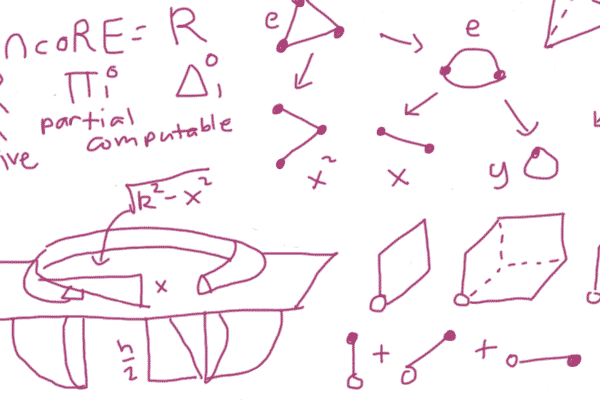Handouts for math contests and other mathy things.

Write-ups and descriptions of some math projects I’ve worked on.

Lecture notes for PRIME, a two-month training program for the Philippine Mathematical Olympiad.

Past problems, answers, and reflections for the PMO, Sipnayan, Mathirang Mathibay, GMATIC.

## Best Of Math

Here’s what I think are some of the best math-related things I’ve written:

### Type Theory by Example

Type theory, focusing on the theory behind proof assistants, with an emphasis on examples. Prerequisites are some familiarity with logic.

### Machine Learning

Machine learning, for people more used to reading math textbooks than writing code. Prerequisites are some knowledge of probability theory, and dealing with matrices and vectors.

### Engineering

Engineering is finding the answer to a problem without solving it. People aren’t trying to engineer enough, so I wrote this to change that.

### Four-Function Primality Testing

Here’s how to use a four-function calculator to check that 123,456,789,011 is prime in fifteen minutes. Discusses the Fermat test and the Miller-Rabin test.

### Obscure Geometry Theorems

Discusses some techniques in computational geometry through the proofs of some lesser-known theorems.

### Crossing Numbers

Talks about the Euler characteristic, Kuratowski’s and Wagner’s theorems, Fáry’s theorem, and the crossing number inequality. No prerequisites, but knowledge of graph theory is useful.